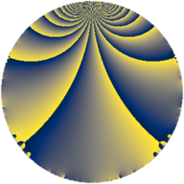# Properties

 Label 342.2.aLevel $342$ Weight $2$ Character orbit 342.a Rep. character $\chi_{342}(1,\cdot)$ Character field $\Q$ Dimension $7$ Newform subspaces $7$ Sturm bound $120$ Trace bound $11$

# Related objects

## Defining parameters

 Level: $$N$$ $$=$$ $$342 = 2 \cdot 3^{2} \cdot 19$$ Weight: $$k$$ $$=$$ $$2$$ Character orbit: $$[\chi]$$ $$=$$ 342.a (trivial) Character field: $$\Q$$ Newform subspaces: $$7$$ Sturm bound: $$120$$ Trace bound: $$11$$ Distinguishing $$T_p$$: $$5$$, $$7$$, $$11$$

## Dimensions

The following table gives the dimensions of various subspaces of $$M_{2}(\Gamma_0(342))$$.

Total New Old
Modular forms 68 7 61
Cusp forms 53 7 46
Eisenstein series 15 0 15

The following table gives the dimensions of the cuspidal new subspaces with specified eigenvalues for the Atkin-Lehner operators and the Fricke involution.

$$2$$$$3$$$$19$$FrickeDim.
$$+$$$$+$$$$+$$$$+$$$$1$$
$$+$$$$-$$$$+$$$$-$$$$2$$
$$+$$$$-$$$$-$$$$+$$$$1$$
$$-$$$$+$$$$+$$$$-$$$$1$$
$$-$$$$-$$$$-$$$$-$$$$2$$
Plus space$$+$$$$2$$
Minus space$$-$$$$5$$

## Trace form

 $$7q - q^{2} + 7q^{4} + 2q^{5} + 2q^{7} - q^{8} + O(q^{10})$$ $$7q - q^{2} + 7q^{4} + 2q^{5} + 2q^{7} - q^{8} + 2q^{10} + 4q^{11} - 6q^{13} + 4q^{14} + 7q^{16} - 4q^{17} - q^{19} + 2q^{20} + 4q^{22} + 10q^{23} - 7q^{25} + 8q^{26} + 2q^{28} - 2q^{29} - 4q^{31} - q^{32} + 2q^{34} + 12q^{35} - 18q^{37} + 3q^{38} + 2q^{40} - 18q^{41} + 8q^{43} + 4q^{44} + 4q^{46} - 20q^{47} - 7q^{49} - 15q^{50} - 6q^{52} + 6q^{53} - 8q^{55} + 4q^{56} - 8q^{58} - 28q^{59} - 10q^{61} + 4q^{62} + 7q^{64} - 8q^{65} + 4q^{67} - 4q^{68} - 12q^{70} + 12q^{71} + 20q^{73} - 10q^{74} - q^{76} - 28q^{77} + 4q^{79} + 2q^{80} - 6q^{82} + 28q^{83} - 24q^{85} - 8q^{86} + 4q^{88} + 26q^{89} + 8q^{91} + 10q^{92} - 8q^{94} - 2q^{95} - 2q^{97} - q^{98} + O(q^{100})$$

## Decomposition of $$S_{2}^{\mathrm{new}}(\Gamma_0(342))$$ into newform subspaces

Label Dim. $$A$$ Field CM Traces A-L signs $q$-expansion
$$a_2$$ $$a_3$$ $$a_5$$ $$a_7$$ 2 3 19
342.2.a.a $$1$$ $$2.731$$ $$\Q$$ None $$-1$$ $$0$$ $$-2$$ $$0$$ $$+$$ $$+$$ $$+$$ $$q-q^{2}+q^{4}-2q^{5}-q^{8}+2q^{10}-2q^{11}+\cdots$$
342.2.a.b $$1$$ $$2.731$$ $$\Q$$ None $$-1$$ $$0$$ $$-2$$ $$0$$ $$+$$ $$-$$ $$+$$ $$q-q^{2}+q^{4}-2q^{5}-q^{8}+2q^{10}+4q^{11}+\cdots$$
342.2.a.c $$1$$ $$2.731$$ $$\Q$$ None $$-1$$ $$0$$ $$0$$ $$-4$$ $$+$$ $$-$$ $$-$$ $$q-q^{2}+q^{4}-4q^{7}-q^{8}-4q^{13}+4q^{14}+\cdots$$
342.2.a.d $$1$$ $$2.731$$ $$\Q$$ None $$-1$$ $$0$$ $$4$$ $$3$$ $$+$$ $$-$$ $$+$$ $$q-q^{2}+q^{4}+4q^{5}+3q^{7}-q^{8}-4q^{10}+\cdots$$
342.2.a.e $$1$$ $$2.731$$ $$\Q$$ None $$1$$ $$0$$ $$0$$ $$-1$$ $$-$$ $$-$$ $$-$$ $$q+q^{2}+q^{4}-q^{7}+q^{8}+6q^{11}+5q^{13}+\cdots$$
342.2.a.f $$1$$ $$2.731$$ $$\Q$$ None $$1$$ $$0$$ $$0$$ $$4$$ $$-$$ $$-$$ $$-$$ $$q+q^{2}+q^{4}+4q^{7}+q^{8}-4q^{11}+4q^{14}+\cdots$$
342.2.a.g $$1$$ $$2.731$$ $$\Q$$ None $$1$$ $$0$$ $$2$$ $$0$$ $$-$$ $$+$$ $$+$$ $$q+q^{2}+q^{4}+2q^{5}+q^{8}+2q^{10}+2q^{11}+\cdots$$

## Decomposition of $$S_{2}^{\mathrm{old}}(\Gamma_0(342))$$ into lower level spaces

$$S_{2}^{\mathrm{old}}(\Gamma_0(342)) \cong$$ $$S_{2}^{\mathrm{new}}(\Gamma_0(19))$$$$^{\oplus 6}$$$$\oplus$$$$S_{2}^{\mathrm{new}}(\Gamma_0(38))$$$$^{\oplus 3}$$$$\oplus$$$$S_{2}^{\mathrm{new}}(\Gamma_0(57))$$$$^{\oplus 4}$$$$\oplus$$$$S_{2}^{\mathrm{new}}(\Gamma_0(114))$$$$^{\oplus 2}$$$$\oplus$$$$S_{2}^{\mathrm{new}}(\Gamma_0(171))$$$$^{\oplus 2}$$